Home Practice
For learners and parents For teachers and schools
Textbooks
Full catalogue
Pricing SupportLog in

We think you are located in United States. Is this correct?

# 4.3 Tariff systems

## 4.3 Tariff systems (EMG49)

Tariff
A list or schedule of pre-determined prices for services like trains, busses, and electrical usage.

Tariffs are pre-determined rates that are used to determine how much money you owe for a given service. For example, the total amount of money you owe on your municipal bill is calculated using predetermined tariffs. A tariff usually includes a unit of payment with a unit of measurement - for example cell phone bills are charged in cents or rands per minute (R/minute) and electricity is charged in cents per kilowatt hour or c/kWh. Telkom charges monthly landline rental in Rands per month.

In this section you will work with different tariff systems, learn how to calculate costs using given tariffs and how to draw and interpret graphs of various tariff systems.

### Municipal tariffs (EMG4B)

Municipal tariffs include tariffs for electricity, water and refuse removal. They differ from one city to the next, but generally speaking, the more electricity or water you use in a month, the more expensive it is per unit. Sometimes municipal tariffs are also called “rates”. Your municipal bill also usually includes a monthly property rate based on the municipal value of your house - again, the more your house is worth, the more you will pay in rates.## Worked example 7: Working with tariffs

The City of Durban's Ethekwini Municipality charges the following tariffs for domestic water usage in 2013, for properties valued at more than $$\text{R}\,\text{250 000}$$:

 Price per kilolitre, excluding VAT $$\text{0}$$ $$\text{kl}$$ to $$\text{9}$$ $$\text{kl}$$ $$\text{R}\,\text{9,50}$$ from $$\text{9}$$ $$\text{kl}$$ to $$\text{25}$$ $$\text{kl}$$ $$\text{R}\,\text{11,22}$$ From $$\text{25}$$ $$\text{kl}$$ to $$\text{30}$$ $$\text{kl}$$ $$\text{R}\,\text{14,95}$$ from $$\text{30}$$ $$\text{kl}$$ to $$\text{45}$$ $$\text{kl}$$ $$\text{R}\,\text{23,05}$$ more than $$\text{45}$$ $$\text{kl}$$ $$\text{R}\,\text{25,36}$$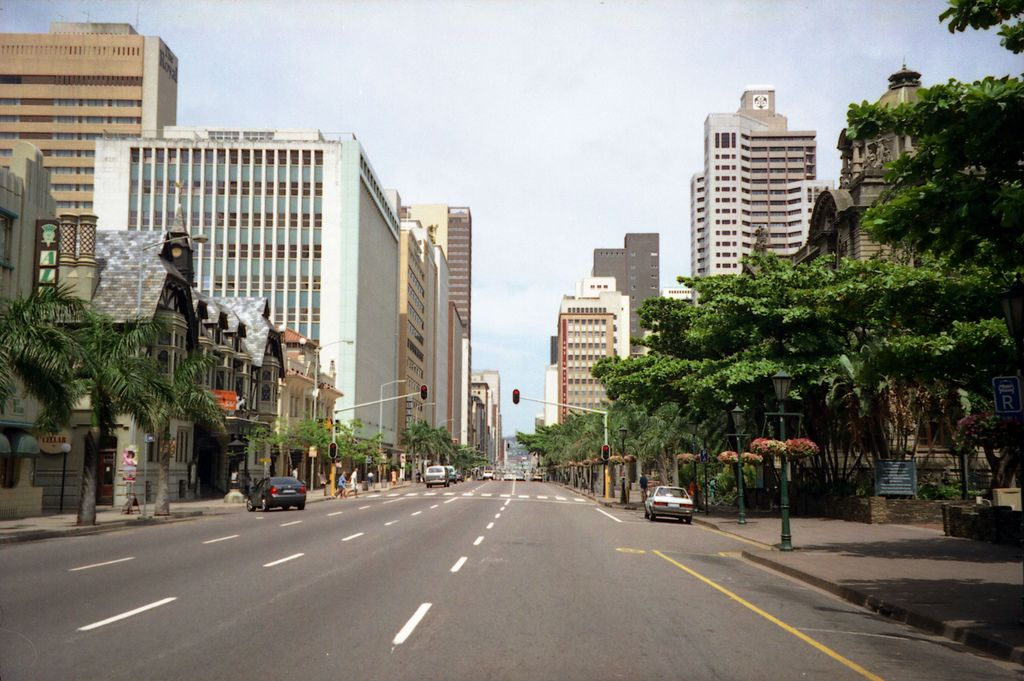1. Megan's monthly water consumption is $$\text{12}$$ $$\text{kl}$$. Calculate her monthly water costs, excluding VAT.

2. Gilbert's monthly water consumption is $$\text{32}$$ $$\text{kl}$$.

1. Calculate what his monthly water bill will be, including VAT.
2. Calculate what Gilbert will pay on average, per kilolitre of water.
3. Study the following graph, showing the relationship between the quantity of water used and the price per unit, as given in the above table: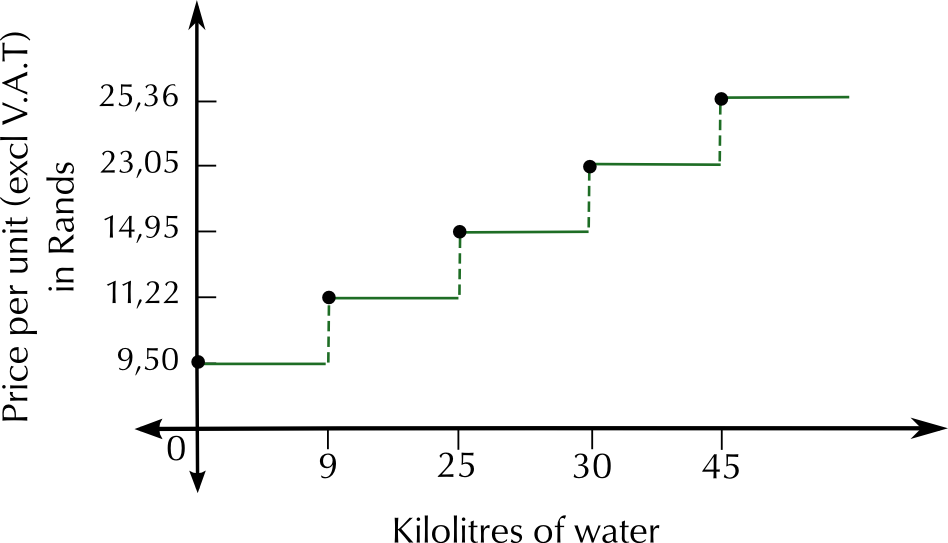Why do you think the graph is a series of horizontal lines and sudden vertical rises?

1. To calculate Megan's total water costs, we break up her usage into the different tariff categories: In the $$\text{0}$$ $$\text{kl}$$ - $$\text{9}$$ $$\text{kl}$$ category, she used $$\text{9}$$ $$\text{kl}$$ of water In the $$\text{9}$$ $$\text{kl}$$ - $$\text{25}$$ $$\text{kl}$$ category, she used $$\text{3}$$ $$\text{kl}$$ of water ($$\text{9}$$ $$\text{kl}$$ + $$\text{2}$$ $$\text{kl}$$ = $$\text{12}$$ $$\text{kl}$$) Now we calculate how much the number of kilolitres used in each category cost: $$\text{9}$$ $$\text{kl}$$ $$\times$$ $$\text{R}\,\text{9,50}$$ = $$\text{R}\,\text{85,50}$$ $$\text{3}$$ $$\text{kl}$$ $$\times$$ $$\text{R}\,\text{11,22}$$ = $$\text{R}\,\text{33,66}$$ Next we add these two subtotals together: $$\text{R}\,\text{85,50}$$ + $$\text{R}\,\text{33,66}$$ = $$\text{R}\,\text{119,16}$$ So her water usage for the month will cost $$\text{R}\,\text{119,16}$$, excluding VAT
1. To calculate Gilbert's total water bill, we break the $$\text{32}$$ $$\text{kl}$$ up into the different tariff categories listed in the table above. In the $$\text{0}$$ $$\text{kl}$$ - $$\text{9}$$ $$\text{kl}$$ category, he used $$\text{9}$$ $$\text{kl}$$ of water In the $$\text{9}$$ $$\text{kl}$$ - $$\text{25}$$ $$\text{kl}$$ category, he used $$\text{16}$$ $$\text{kl}$$ of water ($$\text{9}$$ + $$\text{16}$$ = $$\text{25}$$ $$\text{kl}$$) In the $$\text{25}$$ - $$\text{30}$$ $$\text{kl}$$ category, he used $$\text{5}$$ $$\text{kl}$$ of water ($$\text{25}$$ + $$\text{5}$$ = $$\text{30}$$ $$\text{kl}$$) In the $$\text{30}$$ - $$\text{45}$$ $$\text{kl}$$ category, he used $$\text{2}$$ $$\text{kl}$$ of water ($$\text{30}$$ + $$\text{2}$$ = $$\text{32}$$ $$\text{kl}$$) Now we calculate how much the number of kilolitres used in each category cost: $$\text{9}$$ $$\text{kl}$$ $$\times$$ $$\text{R}\,\text{9,50}$$ = $$\text{R}\,\text{85,50}$$ $$\text{16}$$ $$\text{kl}$$ $$\times$$ $$\text{R}\,\text{11,22}$$ = $$\text{R}\,\text{179,52}$$ $$\text{5}$$ $$\text{kl}$$ $$\times$$ $$\text{R}\,\text{14,95}$$ = $$\text{R}\,\text{74,75}$$ $$\text{2}$$ $$\text{kl}$$ $$\times$$ $$\text{R}\,\text{23,05}$$ = $$\text{R}\,\text{46,10}$$ Next we add these subtotals together to get the total, excluding V.A.T: $$\text{R}\,\text{85,50}$$ + $$\text{R}\,\text{179,52}$$ + $$\text{R}\,\text{74,75}$$ + $$\text{R}\,\text{46,10}$$ = $$\text{R}\,\text{385,87}$$ Lastly, we calculate what the $$\text{14}\%$$ VAT on the above total is, and add it to the total to get the final amount: $$\text{14}\%$$ of $$\text{R}\,\text{385,87}$$ = $$\text{R}\,\text{385,87}$$ $$\times \frac{\text{14}}{\text{100}} =$$$$\text{R}\,\text{54,0218}$$ $$\text{R}\,\text{385,87}$$ + $$\text{R}\,\text{54,0218}$$ $$\approx$$ $$\text{R}\,\text{439,89}$$ So Gilbert's total water costs will be $$\text{R}\,\text{439,89}$$. (Note how we only round down at the last step, to make the calculation more accurate.)
2. Gilbert pays $$\text{R}\,\text{439,89}$$ for $$\text{32}$$ $$\text{kl}$$ of water. $$\frac{\text{R }\text{439,89}}{\text{32}\text{ kl}} \approx \text{R }\text{13,75}$$ per kilolitre.
2. Because the price stays the same from $$\text{0}$$ $$\text{kl}$$ to $$\text{9}$$ $$\text{kl}$$, for example, a horizontal line is drawn between $$\text{0}$$ and $$\text{9}$$. The dotted vertical lines show us how the price suddenly increases when we move into the next group of kilolitres ($$\text{9}$$- $$\text{25}$$ $$\text{kl}$$). The same applies for $$\text{9}$$ - $$\text{25}$$ $$\text{kl}$$, $$\text{25}$$ - $$\text{30}$$ $$\text{kl}$$ etc. We cannot join the points with a sloping line, because this suggests the price per unit rises with each kilolitre, which is not the case, rather the price is constant for a given range of kilolitres (and therefore the graph is flat) and then increases suddenly for each new category of water usage.

## Calculating costs using given municipal tariffs

Exercise 4.5

Domestic electricity in the City of Cape Town is charged for using the tariffs below, for households who use more than $$\text{450}$$ kWh of electricity per month. They refer to each category of electricity usage as a block, and the tariffs are charged in cents per kilowatt hour (kWh). VAT is included in the tariff costs listed below.

 Block number (kWh) cents per kWh (incl VAT) Block 1 ($$\text{0}$$ - $$\text{150}$$ kWh) $$\text{129,05}$$ Block 2 ($$\text{150,1}$$ - $$\text{350}$$ kWh) $$\text{134,65}$$ Block 3 ($$\text{350,1}$$ - $$\text{600}$$ kWh) $$\text{134,65}$$ Block 4 ($$>$$ $$\text{600}$$ kWh) $$\text{159,81}$$

If Jason's household uses $$\text{140}$$ kWh of electricity in a month, calculate what his electricity bill will be, in Rands.

$$\text{140}$$ $$\times$$ $$\text{129,05}$$ $$\text{c}$$ = $$\text{18 067}$$ $$\text{c}$$ = $$\text{R}\,\text{180,67}$$

Thomas uses $$\text{200,5}$$ kWh of electricity in a month. What will his electricity costs be in Rands?

Block $$\text{1}$$:$$\text{140}$$ $$\times$$ $$\text{129,05}$$ $$\text{c}$$ = $$\text{18 067}$$ $$\text{c}$$ = $$\text{R}\,\text{180,67}$$. Block $$\text{2}$$: $$\text{200,5}$$ - $$\text{140}$$ = $$\text{60,5}$$ kWh. $$\text{60,5}$$ $$\times$$ $$\text{134,65}$$ $$\text{c}$$ = $$\text{8 146,325}$$ $$\text{c}$$ = $$\text{R}\,\text{81,46325}$$. $$\text{R}\,\text{180,67}$$ + $$\text{R}\,\text{81,4572}$$ = $$\text{R}\,\text{262,13325}$$ $$\approx$$ $$\text{R}\,\text{262,13}$$

The City of Cape Town decides to introduce a fixed additional tariff for anyone who uses more than $$\text{350}$$ kWh of electricity. This fixed cost is $$\text{R}\,\text{24,45}$$ per month. (I.e. the cost is $$\text{R}\,\text{24,45}$$ plus the price per units used). If Neil uses $$\text{423}$$ kWh of electricity in a month, calculate what his total electricity cost will be.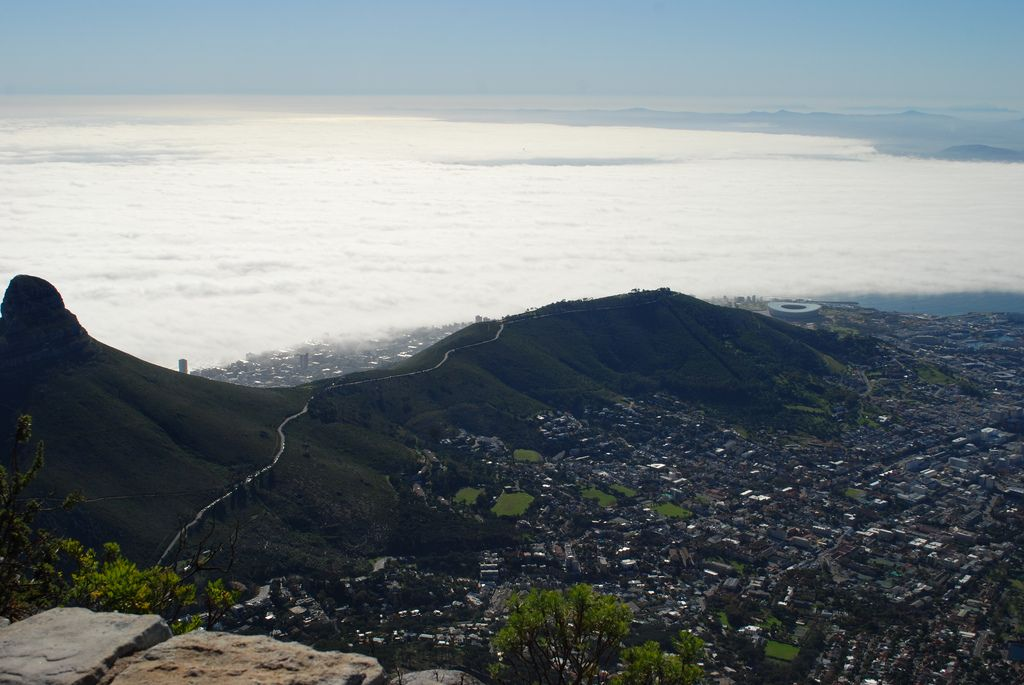Basic cost: $$\text{R}\,\text{24,45}$$. Block $$\text{1}$$:$$\text{140}$$ $$\times$$ $$\text{129,05}$$ $$\text{c}$$ = $$\text{18 067}$$ $$\text{c}$$ = $$\text{R}\,\text{180,67}$$. Block $$\text{2}$$: $$\text{350}$$ - $$\text{150,1}$$ = $$\text{199,99}$$ kWh. $$\text{199,99}$$ $$\times$$ $$\text{134,65}$$ $$\text{c}$$ = $$\text{26 928,6535}$$ $$\text{c}$$ = $$\text{R}\,\text{269,286535}$$. Block $$\text{3}$$: $$\text{423}$$ kWh - $$\text{350,1}$$ kWh = $$\text{72,9}$$ kWh. $$\text{72,9}$$ $$\times$$ $$\text{134,65}$$ $$\text{c}$$ = $$\text{9 815,985}$$ $$\text{c}$$ = $$\text{R}\,\text{98,15985}$$. $$\text{R}\,\text{24,45}$$ + $$\text{R}\,\text{180,67}$$ + $$\text{R}\,\text{269,286535}$$ + $$\text{R}\,\text{98,15985}$$ = $$\text{R}\,\text{572,566385}$$ $$\approx$$ $$\text{R}\,\text{572,57}$$.

### Telephone tariffs (EMG4C)

Fixed phone line and cell phone service providers also commonly charge you for calls using tariffs. The tariff cost can be influenced by factors like the distance of the call (e.g. calls overseas are always more expensive than local calls) and the time of day (most service providers offer cheaper rates in the evenings, depending on which phone package you use).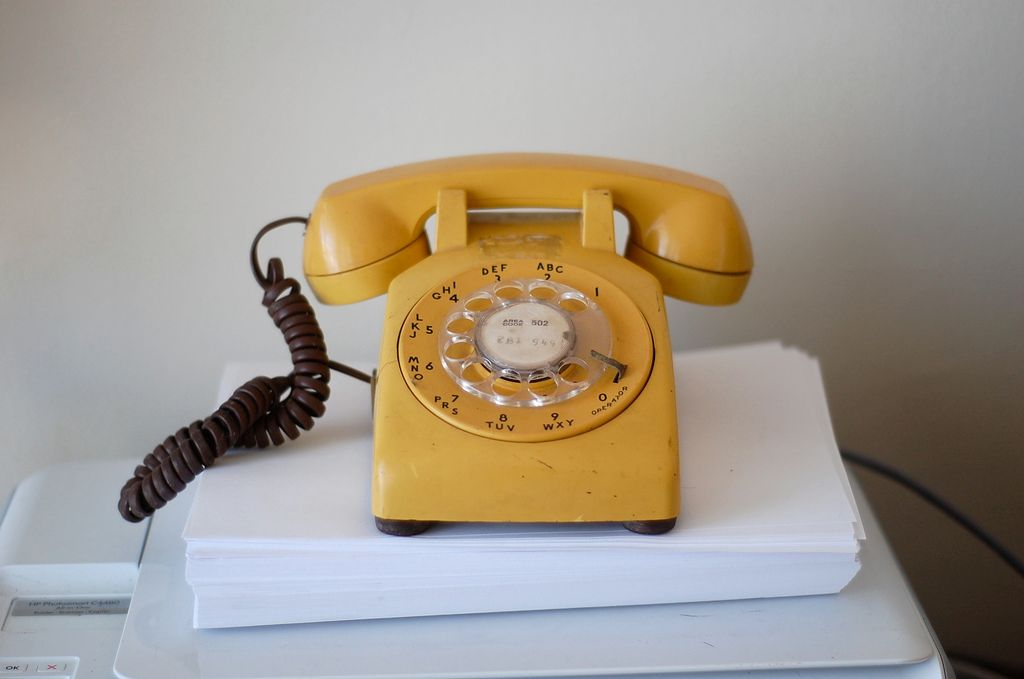## Worked example 8: Working with telephone tariffs

Telkom charges for normal, national landline calls according to the following tariffs:

 Distance Basic, fixed charge in Rands (incl. VAT) (regardless of length of call or distance) Rands per second (incl. VAT) Rands per second (incl. VAT) All times Standard Time: Mon - Fri 07:00 to 19:00 Callmore time: Mon to Fri 19:00 - 7:00 and Fri 19:00 - Mon 7:00 Local ($$\text{0}$$ - $$\text{50}$$ $$\text{km}$$) $$\text{0,570}$$ $$\text{0,00700}$$ $$\text{0,00344}$$ Long distance ($$>$$$$\text{50}$$ $$\text{km}$$) $$\text{0,570}$$ $$\text{0,00950}$$ $$\text{0,00475}$$
1. What two factors can influence the cost of a landline call, based on the information above?
2. Davina calls her mother, who lives $$\text{25}$$ $$\text{km}$$ away, during standard time.

1. If she talks for $$\text{1 000}$$ seconds, what will she pay for the call, in Rands?
2. For how many minutes did they talk? (Round your answer down to the nearest minute).
3. Graham calls his aunt, who lives $$\text{100}$$ $$\text{km}$$ away, during Callmore time. They talk for $$\text{5}$$ minutes. How much will the call cost him?
4. Karabo calls his friend at 5 p.m. on a Saturday. They chat for $$\text{10}$$ minutes. If his friend lives $$\text{5}$$ $$\text{km}$$ away, calculate how much the call with cost Karabo.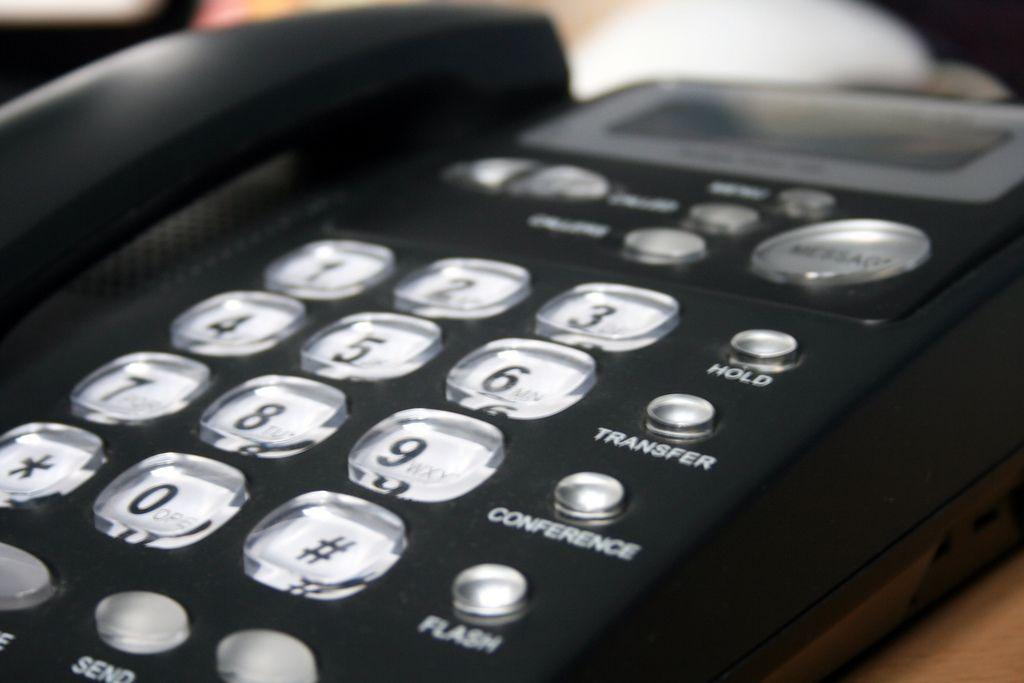1. The distance of the call, and the duration (number of seconds)
1. Cost of call = minimum charge + (Rands per second) $$\times$$ (number of seconds) = $$\text{R}\,\text{0,570}$$ + ($$\text{R}\,\text{0,00700}$$) $$\times$$ $$\text{1 000}$$ =$$\text{R}\,\text{0,570}$$ + $$\text{R}\,\text{7,00}$$ =$$\text{R}\,\text{7,57}$$
2. $$\text{1 000}$$ seconds $$\div$$ $$\text{60}$$ seconds $$\approx$$ $$\text{16}$$ minutes
2. Cost of call = minimum charge + (Rands per second) $$\times$$ (number of seconds) = $$\text{R}\,\text{0,570}$$ + ($$\text{R}\,\text{0,00475}$$) $$\times$$ ($$\text{5}$$ minutes) = $$\text{R}\,\text{0,570}$$ + ($$\text{R}\,\text{0,00475}$$) $$\times$$ ($$\text{5}$$ minutes $$\times$$ $$\text{60}$$) = $$\text{R}\,\text{0,570}$$ + ($$\text{R}\,\text{0,00475}$$) $$\times$$ ($$\text{300}$$ seconds) = $$\text{R}\,\text{0,570}$$ + $$\text{R}\,\text{1,425}$$ = $$\text{R}\,\text{1,995}$$ $$\approx$$ $$\text{R}\,\text{2,00}$$
3. 5 p.m. on a Saturday is during Callmore time, therefore we use the tariffs given in the last column of the table. They chat for $$\text{10}$$ minutes which is $$\text{10}$$ minutes $$\times$$ $$\text{60}$$ = $$\text{600}$$ seconds. Cost of call = minimum charge + (Rands per second) $$\times$$ (number of seconds) = $$\text{R}\,\text{0,570}$$ + ($$\text{R}\,\text{0,00475}$$) $$\times$$ ($$\text{600}$$ seconds) = $$\text{R}\,\text{0,570}$$ + $$\text{R}\,\text{2,85}$$ = $$\text{R}\,\text{3,42}$$

## Interpreting and comparing graphs of a tariff system

Exercise 4.6

A local cellular provider charges the following for a standard contract:

• Monthly subscription: $$\text{R}\,\text{100}$$

• Mandatory itemised billing: $$\text{R}\,\text{22}$$

This monthly contract includes $$\text{R}\,\text{140}$$ worth of airtime and $$\text{R}\,\text{40}$$ worth of free, local SMS's.

Calls and SMS's are charged for using the tariffs given below. This service provider uses per second billing, so the tariff is Rands per $$\text{60}$$ seconds of call time, even if those $$\text{60}$$ seconds are split over two or more calls. Assume that these tariffs, or rates, and the monthly subscription are VAT inclusive. (An SMS is a text message, and an MMS is a text and data message, that may include a photo, for example).

 Rate per minute for the first $$\text{5}$$ minutes of the day $$\text{R}\,\text{1,95}$$ Rate per minute ($$\text{60}$$ seconds) thereafter $$\text{R}\,\text{1,55}$$ Calls to same network $$\text{R}\,\text{0,99}$$ per $$\text{60}$$ seconds International SMS $$\text{R}\,\text{1,20}$$ per SMS SMS $$\text{R}\,\text{0,60}$$ per SMS MMS $$\text{R}\,\text{0,75}$$ per MMS

Alfred has a contract like the one above. Assuming he does not use more than $$\text{R}\,\text{140}$$ airtime in a month and $$\text{R}\,\text{40}$$ worth of SMS's, what will his monthly cell phone bill cost?

Monthly subscription + itemised billing = $$\text{R}\,\text{122}$$

On the first day of the month, the first call Alfred makes is to his father (on a different network) and they talk for $$\text{2}$$ minutes. How much will this call cost Alfred?

$$\text{R}\,\text{1,95}$$ $$\times$$ $$\text{2}$$ = $$\text{R}\,\text{3,90}$$

On the same day, Alfred then calls his friend, Ivan. They talk for $$\text{4}$$ minutes. How much will this second call cost him?

After the first call, Alfred has used $$\text{2}$$ of the first $$\text{5}$$ minutes of calls. The next call will cost ($$\text{3}$$ $$\times$$ $$\text{R}\,\text{1,95}$$) + ($$\text{1}$$ $$\times$$ $$\text{R}\,\text{1,55}$$) = $$\text{R}\,\text{7,40}$$

Later that afternoon, Alfred checks his phone and sees he has made $$\text{9}$$ minutes, $$\text{25}$$ seconds worth of calls.

How many seconds is this?

$$\text{9}$$ minutes $$\text{25}$$ seconds = ($$\text{9}$$ $$\times$$ $$\text{60}$$ seconds) + $$\text{25}$$ seconds = $$\text{565}$$ seconds

If he now calls his friend Azra, who is on the same network, and they talk for $$\text{360}$$ seconds, how much will the call cost him?

$$\text{360}$$ seconds = $$\text{6}$$ $$\times$$ $$\text{60}$$ seconds. Calls to the same network are $$\text{R}\,\text{0,99}$$ per $$\text{60}$$ seconds so it will cost $$\text{R}\,\text{0,99}$$ $$\times$$ $$\text{6}$$ = $$\text{R}\,\text{5,94}$$

Two weeks later, Alfred has made $$\text{R}\,\text{70,45}$$ worth of calls, sent $$\text{25}$$ local SMS's, $$\text{5}$$ international SMS's and $$\text{2}$$ MMS's. Calculate how much airtime he has left.

Alfred starts with $$\text{R}\,\text{140}$$ worth of airtime. We know he has spent $$\text{R}\,\text{70,45}$$ on calls. The local sms's are covered by his monthly contract so we do not deduct them from his airtime (he will have $$\text{15}$$ free local sms's left.) The $$\text{5}$$ international sms's cost $$\text{5}$$ $$\times$$ $$\text{R}\,\text{1,20}$$ = $$\text{R}\,\text{6,00}$$ and the $$\text{2}$$ MMS's cost $$\text{2}$$ $$\times$$ $$\text{R}\,\text{0,75}$$ =$$\text{R}\,\text{1,50}$$. Now we deduct all these costs from the initial amount or $$\text{R}\,\text{140}$$: $$\text{R}\,\text{140}$$ - $$\text{R}\,\text{70,45}$$ - $$\text{R}\,\text{6,00}$$ - $$\text{R}\,\text{1,50}$$ = $$\text{R}\,\text{62,05}$$. He has $$\text{R}\,\text{62,05}$$ airtime left.

Alfred wants to buy an SMS bundle that he thinks is a good deal. With this bundle, he will get $$\text{125}$$ SMS's for an extra $$\text{R}\,\text{78,75}$$ per month (over and above the $$\text{40}$$ free SMS's he already gets).

How much will each of the $$\text{125}$$ SMS's in the new bundle cost him?

$$\text{R}\,\text{0,63}$$

Is this a better deal than what he currently pays for his non-free SMS's?

No. SMS's currently cost him only $$\text{R}\,\text{0,60}$$, so the bundled SMS's are more expensive.

Alfred calls his sister for a big chat, and accidentally uses all of his $$\text{R}\,\text{140}$$ airtime in one day (assume this happened at the beginning of the month and he did not send any SMS's). If she is on a different network, how long did they talk for? Round your answer to the nearest minute, and write it in hours and minutes.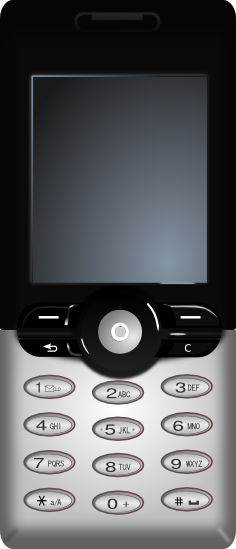The first $$\text{5}$$ minutes cost: $$\text{R}\,\text{1,95}$$ $$\times$$ $$\text{5}$$ = $$\text{R}\,\text{9,75}$$. This leaves him with $$\text{R}\,\text{140}$$ - $$\text{R}\,\text{9,75}$$ = $$\text{R}\,\text{130,25}$$ airtime. The remaining minutes will cost $$\text{R}\,\text{1,55}$$ each. $$\text{R}\,\text{130,25}$$ $$\div$$ $$\text{R}\,\text{1,55}$$ = $$\text{84}$$ minutes = $$\text{1}$$ hour and $$\text{24}$$ minutes.

### Transport tariffs (EMG4D)

Transport tariffs work much the same as municipal and phone tariffs. They may apply to train travel, busses and taxis. Generally, transport tariffs are charged per unit of distance, and the further you need to travel, the higher the tariff and therefore the more expensive your ticket. Like some basic foods, transport fares for taxis, buses and trains in South Africa are exempt from VAT.

## Worked example 9: Working with transport tariffs

The municipal MyCiTi bus system in Cape Town charges the fares below. For all routes except the Airport route, you need to have a myconnect card. For the airport route, you can still use paper tickets, which you can buy at the bus stops at either end of the route.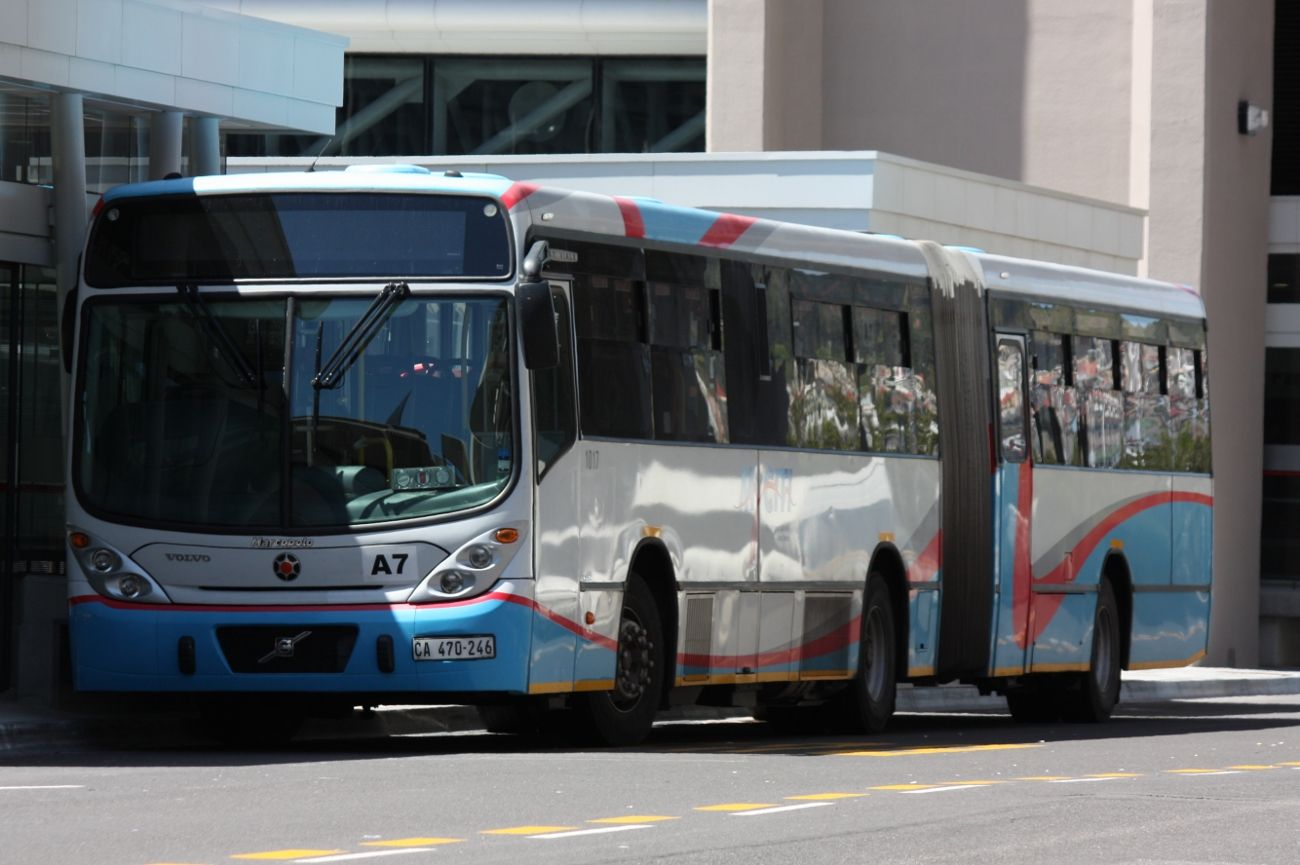Cost Route name $$\text{R}\,\text{23}$$ myconnect card $$\text{R}\,\text{5,30}$$ Gardens - Civic Centre - Waterfront Big Bay - Table View - Parklands East Parklands East - Table View - Blouberg Sands Marine Circle - Table View - Blouberg Sands F1 F14 F15 F16 $$\text{R}\,\text{10,60}$$ Table View - Civic Centre T1 Free Children under $$\text{1}$$ $$\text{m}$$ tall and under $$\text{4}$$ years old (all routes)

Airport Service

 Cost Route name $$\text{R}\,\text{57}$$ Civic Centre - Airport route A1 $$\text{R}\,\text{28,10}$$ Civic Centre - Airport route for children 4 - 11 years old A1 Free Children under 1 metre tall and under $$\text{4}$$ years old A1 $$\text{R}\,\text{499,50}$$ Monthly ticket offering unlimited travel on the Airport - Civic Centre route (A1). This ticket cannot be used by anyone else and must have the name of the ticket holder on it. Write your name on the ticket as soon as buy it. A1
1. Marissa lives near the Waterfront and goes to school in Gardens. She decides to investigate the MyCiTi bus system, route F1.
1. What will the bus ride from the Waterfront to Gardens cost her the first time, and why?
2. After her first bus trip, how much will the journey cost, per trip?
3. If she makes $$\text{10}$$ trips a week ($$\text{5}$$ days to school and back again) what will she be paying per week?
4. Marissa's mother currently drives her to and from school and estimates that she spends $$\text{R}\,\text{13,62}$$ on petrol per day, just for school lifts.

1. How much is Marissa's mother spending on petrol for school lifts per week?
2. How much money will Marissa save her mother by using the MyCiTi bus instead of travelling by car?
2. Thuso's family wants to take the bus from the Civic Centre to Tableview, to go to the beach for the day. He is going with his parents and his aunt, his younger sister and his twin brothers who are $$\text{3}$$ years old. How much will a one way trip to the beach cost the family, if both Thuso and his sister (who are both teenagers) have myconnect cards but no one else in the family does?
3. Karen lives in Johannesburg but regularly travels to Cape Town for work. Her Cape Town office is based near the Civic Centre, so it is convenient to catch the MyCiTi bus from the airport to the Civic Centre in town.
1. How much would a single trip from the airport to the Civic Centre cost her?
2. On average, Karen makes $$\text{5}$$ round trips (from the airport to the Civic Centre and back again) in a month.

1. Is there a cheaper bus fare or package she could use?
2. If she buys the unlimited monthly Airport ticket, how much will each trip cost her? Is this cheaper than buying single trip tickets?
1. She has to buy a myconnect card to use the bus, so the first trip will cost her $$\text{R}\,\text{23,00}$$ (for the card) + $$\text{R}\,\text{5,30}$$ (for the bus ride) or $$\text{R}\,\text{28,30}$$ in total.
2. Thereafter it will only cost her $$\text{R}\,\text{5,30}$$ per trip.
3. $$\text{R}\,\text{5,30}$$ $$\times$$ $$\text{2}$$ trips per day $$\times$$ $$\text{5}$$ = $$\text{R}\,\text{53,00}$$ per week
1. $$\text{R}\,\text{13,62}$$ $$\times$$ $$\text{5}$$ days = $$\text{R}\,\text{68,10}$$ per week
2. $$\text{R}\,\text{68,10}$$ - $$\text{R}\,\text{53,00}$$ = $$\text{R}\,\text{15,10}$$. Marissa will be saving her mother $$\text{R}\,\text{15,10}$$ per week.
1. Thuso's parents and aunt will all need to buy myconnect cards, so their fares will cost $$\text{3}$$ $$\times$$ ($$\text{R}\,\text{23,00}$$ + $$\text{R}\,\text{10,60}$$) = $$\text{3}$$ $$\times$$ $$\text{R}\,\text{33,60}$$ = $$\text{R}\,\text{100,80}$$ Thuso and his sister already have cards, so they only pay for the trip: $$\text{2}$$ $$\times$$ $$\text{R}\,\text{10,60}$$ = $$\text{R}\,\text{21,20}$$ His baby brothers travel for free because they are under the age of $$\text{4}$$. So the total cost is: $$\text{R}\,\text{100,80}$$ + $$\text{R}\,\text{21,20}$$ = $$\text{R}\,\text{122,00}$$.
1. $$\text{R}\,\text{57,00}$$
1. Yes - she could investigate the monthly, unlimited travel option between the Airport and the Civic Centre.
2. The monthly rate is $$\text{R}\,\text{499,50}$$. $$\text{5}$$ round trips = $$\text{10}$$ one way trips. $$\text{R}\,\text{499,50}$$ $$\div$$ $$\text{10}$$ = $$\text{R}\,\text{49,95}$$ per trip. This is much cheaper than $$\text{R}\,\text{57}$$ per trip!

## Working with transport tariffs

Exercise 4.7

Metrorail in Gauteng charges the following tariffs for Metro (Standard) Class tickets for different zones:

 Zone Single Return Weekly Monthly $$\text{1}$$ - $$\text{19}$$ $$\text{km}$$ $$\text{4,00}$$ $$\text{7,50}$$ $$\text{22,00}$$ $$\text{81,50}$$ $$\text{20}$$ - $$\text{29}$$ $$\text{km}$$ $$\text{5,00}$$ $$\text{9,50}$$ $$\text{27,50}$$ $$\text{97,00}$$ $$\text{30}$$ - $$\text{39}$$ $$\text{km}$$ $$\text{6,00}$$ $$\text{11,50}$$ $$\text{32,50}$$ $$\text{112,00}$$ $$\text{40}$$ - $$\text{49}$$ $$\text{km}$$ $$\text{7,50}$$ $$\text{14,50}$$ $$\text{34,00}$$ $$\text{123,00}$$ $$>$$ $$\text{50}$$ $$\text{km}$$ $$\text{9,50}$$ $$\text{18,50}$$ $$\text{38,50}$$ $$\text{140,00}$$

Chuma travels $$\text{15}$$ $$\text{km}$$ on the train every day to school and back again. She buys a single ticket for every trip that she makes.

how many trips will Chuma make (to school and back) in one month ($$\text{4}$$ weeks)?

$$\text{2}$$ trips per day $$\times$$ $$\text{5}$$ school days per week $$\times$$ $$\text{4}$$ weeks = $$\text{40}$$ trips per month

How much will this cost her, if she buys single tickets?

$$\text{40}$$ $$\times$$ $$\text{R}\,\text{4,00}$$ = $$\text{R}\,\text{160,00}$$

How much cheaper will a monthly ticket be?

$$\text{R}\,\text{160,00}$$ - $$\text{R}\,\text{81,50}$$ = $$\text{R}\,\text{78,50}$$ cheaper.

If she buys a monthly ticket for $$\text{R}\,\text{81,50}$$, how much will each trip cost her?

$$\text{R}\,\text{81,50}$$ $$\div$$ $$\text{40}$$ trips = $$\text{R}\,\text{2,04}$$.

How much cheaper than a single ticket is this?

$$\text{R}\,\text{4,00}$$ - $$\text{R}\,\text{2,04}$$ = $$\text{R}\,\text{1,96}$$ cheaper.

Lindiwe has a monthly ticket and travels a distance of $$\text{35}$$ $$\text{km}$$, return, every day.

How much does her monthly ticket cost her?

$$\text{R}\,\text{112,00}$$

Lindiwe's friend tells her that it's possible to get a $$\text{20}\%$$ discount if you're a scholar, wearing your school uniform. What would her monthly ticket cost with a $$\text{20}\%$$ discount?$$\text{R}\,\text{112,00}$$ $$\times$$ $$\text{0,20}$$ = $$\text{R}\,\text{22,40}$$. $$\text{R}\,\text{112,00}$$ - $$\text{R}\,\text{22,40}$$ = $$\text{R}\,\text{89,60}$$